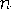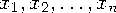Functions and CALL Routines

# HARMEAN Function

Returns the harmonic mean.
 Category: Descriptive Statistics

## Syntax

 HARMEAN(argument<,argument,...>)

### Arguments

argument

is a non-negative numeric constant, variable, or expression.

 Tip: The argument list can consist of a variable list, which is preceded by OF.

The MEAN function returns the arithmetic mean (average), and the GEOMEAN function returns the geometric mean, whereas the HARMEAN function returns the harmonic mean of the non-missing values. Unlike HARMEANZ, HARMEAN fuzzes the values of the arguments that are approximately zero.

If any argument is negative, then the result is a missing value. A message appears in the log that the negative argument is invalid, and _ERROR_ is set to 1. If all the arguments are missing values, then the result is a missing value. Otherwise, the result is the harmonic mean of the non-missing values.

If any argument is zero, then the harmonic mean is zero. Otherwise, the harmonic mean is the reciprocal of the arithmetic mean of the reciprocals of the values.

Letbe the number of arguments with non-missing values, and letbe the values of those arguments. The harmonic mean isFloating-point arithmetic often produces tiny numerical errors. Some computations that result in zero when exact arithmetic is used might result in a tiny non-zero value when floating-point arithmetic is used. Therefore, HARMEAN fuzzes the values of arguments that are approximately zero. When the value of one argument is extremely small relative to the largest argument, then the former argument is treated as zero. If you do not want SAS to fuzz the extremely small values, then use the HARMEANZ function.

SAS Statements Results
`x1=harmean(1,2,4,4);`
`2`
`x2=harmean(.,4,12,24);`
`8`
`x3=harmean(of x1-x2);`
`3.2`

 Function:Previous Page | Next Page | Top of Page